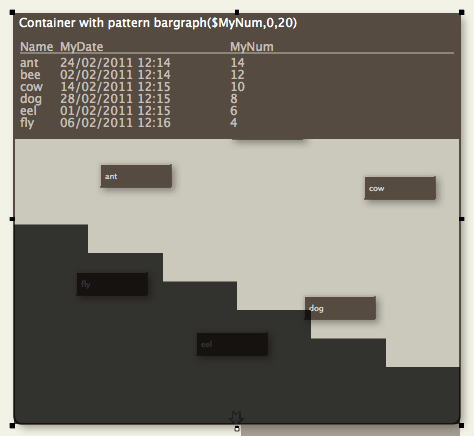# Pattern: bargraph() (for container plot only)Note that this pattern cannot be set via the Interior Inspector, use the Plot Inspector. The pattern requires configuration via its parameters. This pattern can be used in shaped notes. This pattern is only available for use in container plots and has no visual effect if applied to other objects.

The graph is drawn in the form of a bar graph plot across the viewport area of the container. The graph is drawn in colour \$PlotColor. The container viewport is still accessible for drag/drop, etc., as if the plot were not there. Think of the plot as an overlay.

For example, to graph the word count of each child note in the container in bar graph form, set the container's map Pattern attribute to:

`bargraph(\$WordCount)`

The Y-axis of the graph will run from the minimum value of the specified attribute, for the notes being plotted, to the largest value.

The pattern accepts optional minimum and maximum values:

bargraph(\$ValuesSource,min,max)

Thus:

`bargraph(\$WordCount,0)`

graphs the word count of each note whilst ensuring the Y-axis is based at zero, with all attribute values including the maximum being plotted. Note that while zero is the default value of an 'blank' number type attribute, the type allows minus values. The above example would treat all of them as if their value were zero. If a negative min value is supplied, negative item values above that threshold are plotted. For:

`bargraph(\$WordCount,10,900)`

the plot graphs the data from a baseline of 10 to a maximum value of 900. Values outside these are plotted appropriately as the min or max values.

An alternate container plot type is plot() which draws a sparkline-type plot of each child item's value or xyplot() which draws a similar sparkline-type plot but where data can be specified for both axes.

The background of the plot is set via \$PlotBackgroundColor with opacity of \$PlotBackgroundOpacity.

A Tinderbox Reference File : Visual Styling : Shapes, borders, patterns and fills : Pattern: bargraph() (for container plot only)